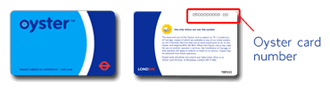Oyster card is a payment card issued by Transport for London for public transport within its network. Every Oyster card has a unique number on its back.The number is in the format of 10 digits, followed by a checksum of 2 digits, both of which may include leading zeros, for example, `0000000000 00`.

With some experiments, I found the checksum can be calculated with the following formula:

 `````` 1 2 3 4 5 6 7 8 9 10 11 `````` ``````def checksum(number: int) -> int: return ( + number * 0x10 + number // 0x1 * 3 - number // 0x10 * 3 + number // 0x100 * 3 - number // 0x1000 * 3 + number // 0x10000 * 3 - number // 0x100000 * 3 + number // 0x1000000 * 3 - number // 0x10000000 * 3) % 100 ``````